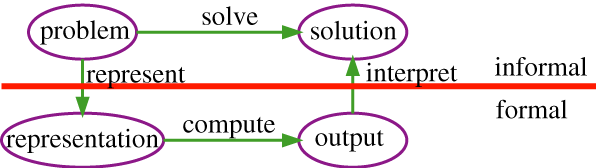## 1.4 Knowledge Representation

Typically, a problem to solve or a task to carry out, as well as what constitutes a solution, is only given informally, such as "deliver parcels promptly when they arrive" or "fix whatever is wrong with the electrical system of the house."Figure 1.4: The role of representations in solving problems

The general framework for solving problems by computer is given in Figure 1.4. To solve a problem, the designer of a system must

• flesh out the task and determine what constitutes a solution;
• represent the problem in a language with which a computer can reason;
• use the computer to compute an output, which is an answer presented to a user or a sequence of actions to be carried out in the environment; and
• interpret the output as a solution to the problem.

Knowledge is the information about a domain that can be used to solve problems in that domain. To solve many problems requires much knowledge, and this knowledge must be represented in the computer. As part of designing a program to solve problems, we must define how the knowledge will be represented. A representation scheme is the form of the knowledge that is used in an agent. A representation of some piece of knowledge is the internal representation of the knowledge. A representation scheme specifies the form of the knowledge. A knowledge base is the representation of all of the knowledge that is stored by an agent.

A good representation scheme is a compromise among many competing objectives. A representation should be

• rich enough to express the knowledge needed to solve the problem.
• as close to the problem as possible; it should be compact, natural, and maintainable. It should be easy to see the relationship between the representation and the domain being represented, so that it is easy to determine whether the knowledge represented is correct. A small change in the problem should result in a small change in the representation of the problem.
• amenable to efficient computation, which usually means that it is able to express features of the problem that can be exploited for computational gain and able to trade off accuracy and computation time.
• able to be acquired from people, data and past experiences.

Many different representation schemes have been designed. Many of these start with some of these objectives and are then expanded to include the other objectives. For example, some are designed for learning and then expanded to allow richer problem solving and inference abilities. Some representation schemes are designed with expressiveness in mind, and then inference and learning are added on. Some schemes start from tractable inference and then are made more natural, and more able to be acquired.

Some of the questions that must be considered when given a problem or a task are the following:

• What is a solution to the problem? How good must a solution be?
• How can the problem be represented? What distinctions in the world are needed to solve the problem? What specific knowledge about the world is required? How can an agent acquire the knowledge from experts or from experience? How can the knowledge be debugged, maintained, and improved?
• How can the agent compute an output that can be interpreted as a solution to the problem? Is worst-case performance or average-case performance the critical time to minimize? Is it important for a human to understand how the answer was derived?

These issues are discussed in the next sections and arise in many of the representation schemes presented later in the book.